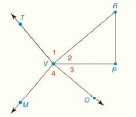Chapter 1.CR, Problem 38CR### Elementary Geometry for College St...

6th Edition
Daniel C. Alexander + 1 other
ISBN: 9781285195698

#### Solutions

Chapter
Section### Elementary Geometry for College St...

6th Edition
Daniel C. Alexander + 1 other
ISBN: 9781285195698
Textbook Problem
12 views

# Fill in the missing statements or reasons. Given: ∠ 1 ≅ ∠ P ∠ 4 ≅ ∠ P V P ¯ bisects ∠ R V O Prove: ∠ T V P ≅ ∠ M V P Proof Statements Reasons 1. ∠ 1 ≅ ∠ P 1. Given 2. ? 2. Given (1), (2) 3. ? 3. Transitive Property of ≅ (3) 4. m ∠ 1 = m ∠ 4 4. ? 5. V P ¯ bisects ∠ R V O 5. ? 6. ? 6. If a ray bisects an ∠ , it forms two ∠ s of equal measure (4), (6), 7. ? 7. Addition Property of Equality 8. m ∠ 1 + m ∠ 2 = m ∠ T V P ; m ∠ 4 + m ∠ 3 = m ∠ M V P ; 8. ? (7), (8) 9. m ∠ T V P = m ∠ M V P 9. ? 10. ? 10. If two ∠ s are = in measure, then they are ≅To determine

To find:

The missing statements for the algebraic proof.

Explanation

Given:

The given diagram is,

The given table is shown below.

 PROOF Statements Reasons 1. ∠1≅∠P 1. Given 2. ? 2. Given (1), (2) 3. ? 3. Transitive Property of ≅ (3) 4. m∠1=m∠4 4. ? 5. VP¯ bisects ∠RVO 5. ? 6. ? 6. If a ray bisects an ∠, it forms two ∠s of equal measure (4), (6), 7. ? 7. Addition Property of Equality 8. m∠1+m∠2=m∠TVP;m∠4+m∠3=m∠MVP; 8. ? (7), (8) 9. m∠TVP=m∠MVP 9. ? 10. ? 10. If two ∠s are = in measure, then they are ≅

1P

4P

VP¯ bisects RVO

Basic properties:

a. The Transitive Property of Congruence states that if two angles are each congruent to a third angle, then they are congruent to each other.

b. The Additive Property of Equality states that if same number is added to both sides of the equation then the sides of the equation remains equal.

c. If the measure of two angles is equal then the angles are known as congruent angles.

d. According to Substitution Property, if x=y, then x can be substituted for y and y can be substituted for x in any equation.

Calculation:

The second statement of the proof is given. That is.

4P

The third statement is of the proof of Transitive Property of Congruence. So, use Transitive Property of Congruence in the given diagram.

14

The fourth reason is based on angles of congruence. According to this, two angles will have same measure if they are congruent.

The fifth statement of the proof is given.

The sixth statement is based on angle bisector. According to this, an angle bisector bisects an angle into two equal angles. That is,

m2=m3

The seventh statement of the proof is Addition Property of Equality. So,

m1+m2=m4+m3

The eighth reason is based on Angle-Addition Property. According to this, if T is the interior of TVP, then,

m1+m2=mTVP;m4+m3=mMVP

The ninth reason is based on Substitution Property.

The tenth statement of the proof is based on the property that two angles are said to be congruent if they are equal in measure.

The table with statements of the reasons is shown below.

 PROOF Statements Reasons 1. ∠1≅∠P 1

### Still sussing out bartleby?

Check out a sample textbook solution.

See a sample solution

#### The Solution to Your Study Problems

Bartleby provides explanations to thousands of textbook problems written by our experts, many with advanced degrees!

Get Started